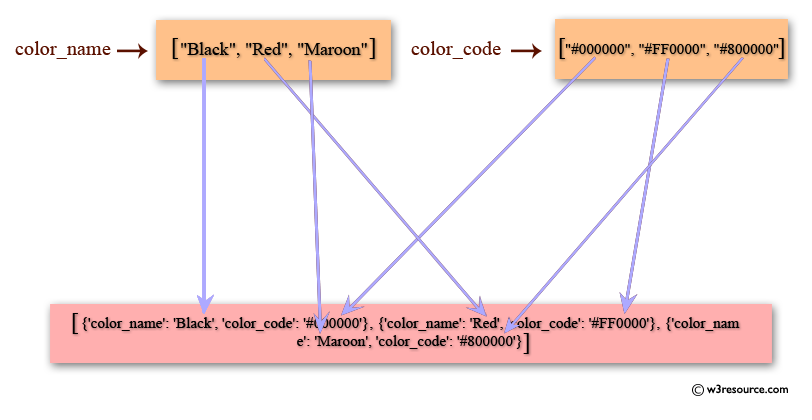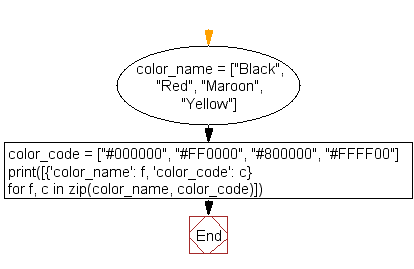﻿ Python: Convert list to list of dictionaries - w3resource# Python: Convert list to list of dictionaries

## Python List: Exercise - 49 with Solution

Write a Python program to convert list to list of dictionaries.Sample Solution:-

Python Code:

``````color_name = ["Black", "Red", "Maroon", "Yellow"]
color_code = ["#000000", "#FF0000", "#800000", "#FFFF00"]
print([{'color_name': f, 'color_code': c} for f, c in zip(color_name, color_code)])
```
```

Sample Output:

```[{'color_name': 'Black', 'color_code': '#000000'}, {'color_name': 'Red', 'color_code': '#FF0000'}, {'color_nam
e': 'Maroon', 'color_code': '#800000'}, {'color_name': 'Yellow', 'color_code': '#FFFF00'}]
```

Flowchart:## Visualize Python code execution:

The following tool visualize what the computer is doing step-by-step as it executes the said program:

Python Code Editor:

Have another way to solve this solution? Contribute your code (and comments) through Disqus.

What is the difficulty level of this exercise?

Test your Python skills with w3resource's quiz

﻿

## Python: Tips of the Day

Floor Division:

When we speak of division we normally mean (/) float division operator, this will give a precise result in float format with decimals.

For a rounded integer result there is (//) floor division operator in Python. Floor division will only give integer results that are round numbers.

```print(1000 // 300)
print(1000 / 300)```

Output:

```3
3.3333333333333335```